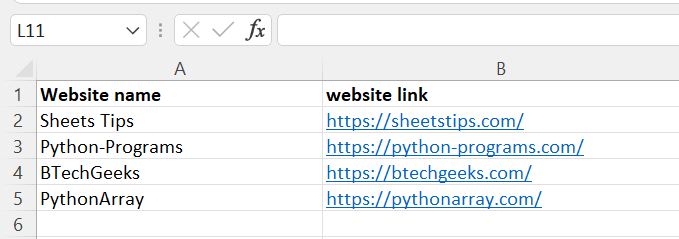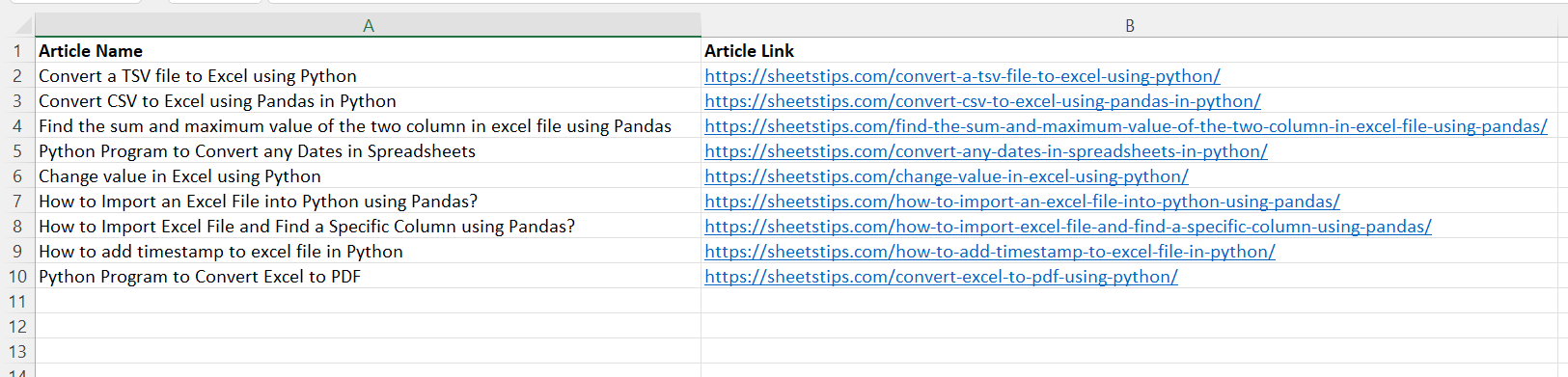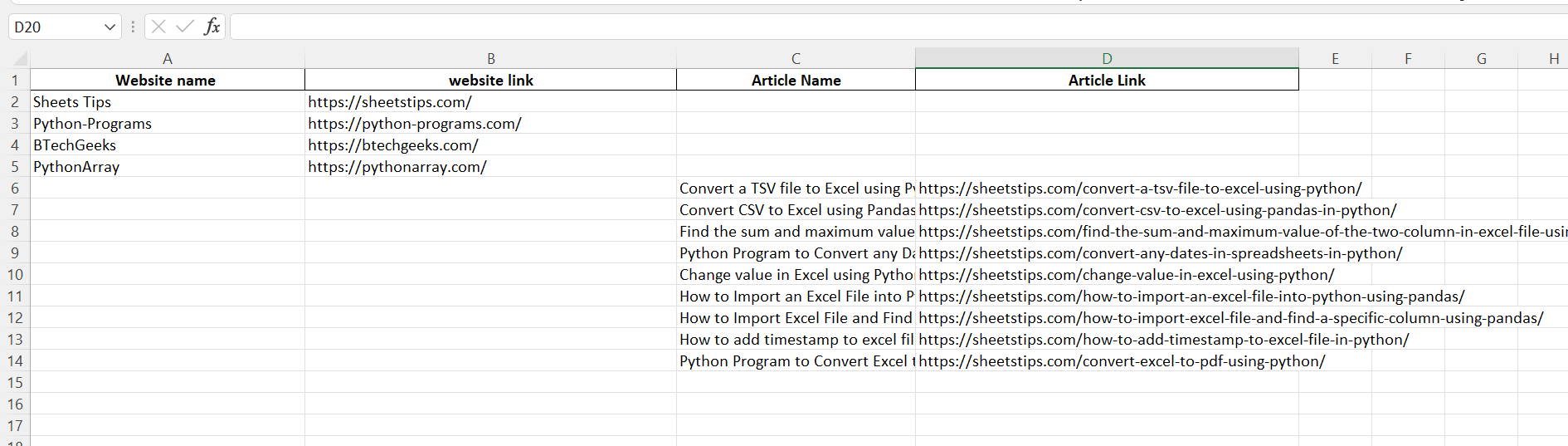# How to Merge all Excel Files in a Folder using Python?

In this article, we are going to merge all the files in a folder using Python.

Prerequisites:

## Python Program to Merge all Excel Files in a Folder

Excel sheet 1(SampleExcel1.xlsx):Excel sheet 2(SampleExcel2.xlsx):We will see the step by step approach for merging all the excel files in a folder in Python:

1)Importing the modules

• Importing the required modules and libraries (pandas and glob) using the import keyword.

Below is the Implementation:

```# Importing the required modules and libraries (pandas and glob) using the import keyword.
import pandas as pd
import glob```

2)Specifying the path of the folder where excel files are stored

• Giving the path of the folder(where the excel files are stored) as static input and storing it in a variable(This piece of code will retrieve the folder containing the files).

Below is the Implementation:

```# Giving the path of the folder(where the excel files are stored) as static input
# and storing it in a variable(This piece of code will retrieve the folder containing the files).
path=r'Excel_Files'```

3)Getting all the Excel Files in the Folder using the glob function

• Pass the path of the folder and extension of the files and concatenate using string concatenation and pass this result as an argument to the glob() function and store them in a variable.
• Print the names of the files obtained from the above result using the print() function.

Below is the Implementation:

```# Pass the path of the folder and extension of the files and concatenate using string concatenation
# and pass this result as an argument to the glob() function and store them in a variable.
file_names = glob.glob(files_path + "\*.xlsx")
# Print the names of the files obtained from the above result using the print() function.
print('The Files which are present in the folder :\n', file_names)
```

4)Initializing Empty DataFrame

• In Python, a Data Frame is a Table data structure used for data analysis and manipulation.
• We take an empty data frame to store the result of the excel files in the folder.
• We get an empty dataframe using the dataframe() function.

Below is the implementation:

```# In Python, a Data Frame is a Table data structure used for data analysis and manipulation.
# We take an empty data frame to store the result of the excel files in the folder.
# We get an empty dataframe using the dataframe() function.
resultExcelFile = pd.DataFrame()```

5)Merging Excel Files

```The read excel method accepts two arguments: sheet name and index col.
sheet name defines the sheet from which the data frame should be created, and the index col gives the title column```

append() function:

```The append() function in Pandas DataFrame is used to merge rows from another DataFrame object.
This function creates a new DataFrame object without changing the source objects.
If the columns do not match, new columns are added to the output DataFrame.```

concat() function:

```Concatenate pandas objects along a specific axis with optional set logic along the other axes
using the concat() function.```
• Iterate through all the excel files in the folder using the For loop.
• Pass the arguments file name, sheet name, etc to the read_excel() function to read the current iterator excel file.
• Add the read_excel file to the concat() method of the pandas module to concatenate the given file and store that pandas dataframe to a variable.
• Append the above pandas dateframe to the resultExcelFile pandas dataframe using the pandas append() function and store that in a same variable.

Below is the Implementation:

```# Iterate through all the excel files in the folder using the For loop.
for file_name in file_names:
# Pass the arguments file name, sheet name, etc to the read_excel() function to read the current iterator excel file.
# Add the read_excel file to the concat() method of the pandas module to
# concatenate the given file and store that pandas dataframe to a variable.

# Append the above pandas dateframe to the resultExcelFile pandas dataframe using the pandas append()
# function and store that in a same variable.
resultExcelFile = resultExcelFile.append(dataframe, ignore_index=True)

```

6)Converting ResultDataFrame to Excel

to_excel() function:

```To export the DataFrame to an excel file, use the to_excel() method. The target file name must
be specified when writing a single object to an excel file. If we wish to write to many sheets,
we must first construct an ExcelWriter object with the target filename and then indicate the
sheet in the file we want to write to. The unique sheet name can also be used to write numerous
sheets. All modifications made to the data written to the file must be saved.```

Below is the Implementation:

• Convert the result data frame to excel using the to_excel() function by passing the result excel path.
```# Convert the result data frame to excel using the to_excel() function by passing the result excel path.
resultExcelFile.to_excel(r'C:\Users\cirus\Desktop\result_excel.xlsx',index=False)
```

### Below is the complete approach for the above program:

Approach:

• Importing the required modules and libraries (pandas and glob) using the import keyword.
• Giving the path of the folder(where the excel files are stored) as static input and storing it in a variable(This piece of code will retrieve the folder containing the files).
• Pass the path of the folder and extension of the files and concatenate using string concatenation and pass this result as an argument to the glob() function and store them in a variable.
• Print the names of the files obtained from the above result using the print() function.
• In Python, a Data Frame is a Table data structure used for data analysis and manipulation.
• We take an empty data frame to store the result of the excel files in the folder.
• We get an empty dataframe using the dataframe() function.
• In Python, a Data Frame is a Table data structure used for data analysis and manipulation.
• We take an empty data frame to store the result of the excel files in the folder.
• We get an empty dataframe using the dataframe() function.
• Iterate through all the excel files in the folder using the For loop.
• Pass the arguments file name, sheet name, etc to the read_excel() function to read the current iterator excel file.
• Add the read_excel file to the concat() method of the pandas module to concatenate the given file and store that pandas dataframe to a variable.
• Append the above pandas dateframe to the resultExcelFile pandas dataframe using the pandas append() function and store that in a same variable.
• Convert the result data frame to excel using the to_excel() function by passing the result excel path.

Below is the full implementation of the code:

```# Importing the required modules and libraries (pandas and glob) using the import keyword.
import pandas as pd
import glob
# Giving the path of the folder(where the excel files are stored) as static input
# and storing it in a variable(This piece of code will retrieve the folder containing the files).
path=r'Excel_Files'
# Pass the path of the folder and extension of the files and concatenate using string concatenation
# and pass this result as an argument to the glob() function and store them in a variable.
file_names = glob.glob(files_path + "\*.xlsx")
# Print the names of the files obtained from the above result using the print() function.
print('The Files which are present in the folder :\n', file_names)
# In Python, a Data Frame is a Table data structure used for data analysis and manipulation.
# We take an empty data frame to store the result of the excel files in the folder.
# We get an empty dataframe using the dataframe() function.
resultExcelFile = pd.DataFrame()
# Iterate through all the excel files in the folder using the For loop.
for file_name in file_names:
# Pass the arguments file name, sheet name, etc to the read_excel() function to read the current iterator excel file.
# Add the read_excel file to the concat() method of the pandas module to
# concatenate the given file and store that pandas dataframe to a variable.